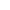Characteristics

• Detection mode:

absorbance

• Applications:

ELISA

Description

Functions

1. It can do self-check in boot-strap.

2. It can select filter automatically.

3. It has the function of vibration.

4. It can communicate and it has two protocols to choose.

5. It can store 126 test-programs, which can be called in test.

6. It can store 126 groups of calibrating parameters and curve, and it also can store 105 groups of testing data for full-plate.

7. It has single and dual wavelength test to be selected by user.

8. It can output ten kinds data.

9. It has the analysis function of absorbance, qualitative and quantitative. Thereinto qualitative test has two methods, which respectively is critical value and critical value adjustment, and you can setup the area of gray scale at will; the quantitative test has nine methods, which respectively is linear regression, semi-logarithm, full-logarithm, exponential regression, ratio regression, quadratic equation, point to point, ratio semi-logarithm and Logit-log curve.

Other Perlove Medical products